Subject:
Mathematics

Topics: AlgebraCoordinate SystemsExpressions and Equations
Common Core State Standard: F-IF.7e,
Knowledge and Skills:

• Can evaluate expressions by substituting values for variables
• Can simplify expressions using correct order of operations
• Can plot a point in a two-dimensional coordinate system, given the coordinates, or determine the coordinates of a given point

Lesson:

If the Space Shuttle were to make a maintenance trip out to a satellite in geosynchronous orbit, the Shuttle would need to enter into an elliptical orbit around the earth. That orbit could be described by this equation: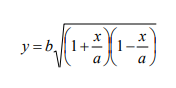where a and b are numbers that determine the shape of the ellipse.

If all distances are measured in thousands of miles, then an actual orbit might be described by this equation: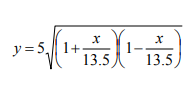1. Find the value of y for each value of x (to two decimal places):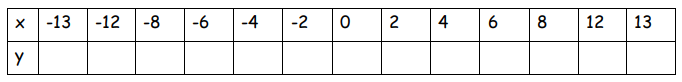2. Plot the ordered pairs from the table above and sketch the curve that
connects them.

3. In this orbit, the Earth would be at the position (-12.5,0), and the satellite
would be at the position (13.5,0). Plot these points on your graph. How far is
the earth from the satellite?

1.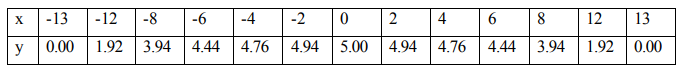2.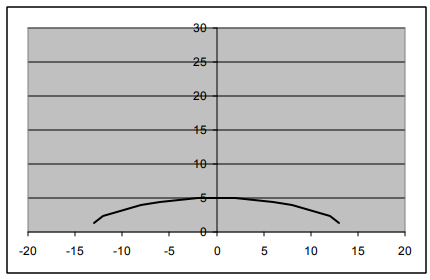3.

26,000 miles